# Monge's Method - Perpendicularity

RECALL basic definitions and theorems on perpendicularity.

### Orthogonal projection of perpendicular straight lines

It was mentioned earlier that  projecting an angle, measure of its projection is NOT the same. Therefore:

• Orthogonal projections of two perpendicular lines are NOT perpendicular lines.

The right angle (and all other angles) will be projected in true size if its sides are parallel to the plane of projection.

The following holds for the right angle:
• Orthogonal projection of the right angle is in true size (also a right angle) if one of its sides is parallel to the plane of projection.

Proof of this statement can be seen on the image below.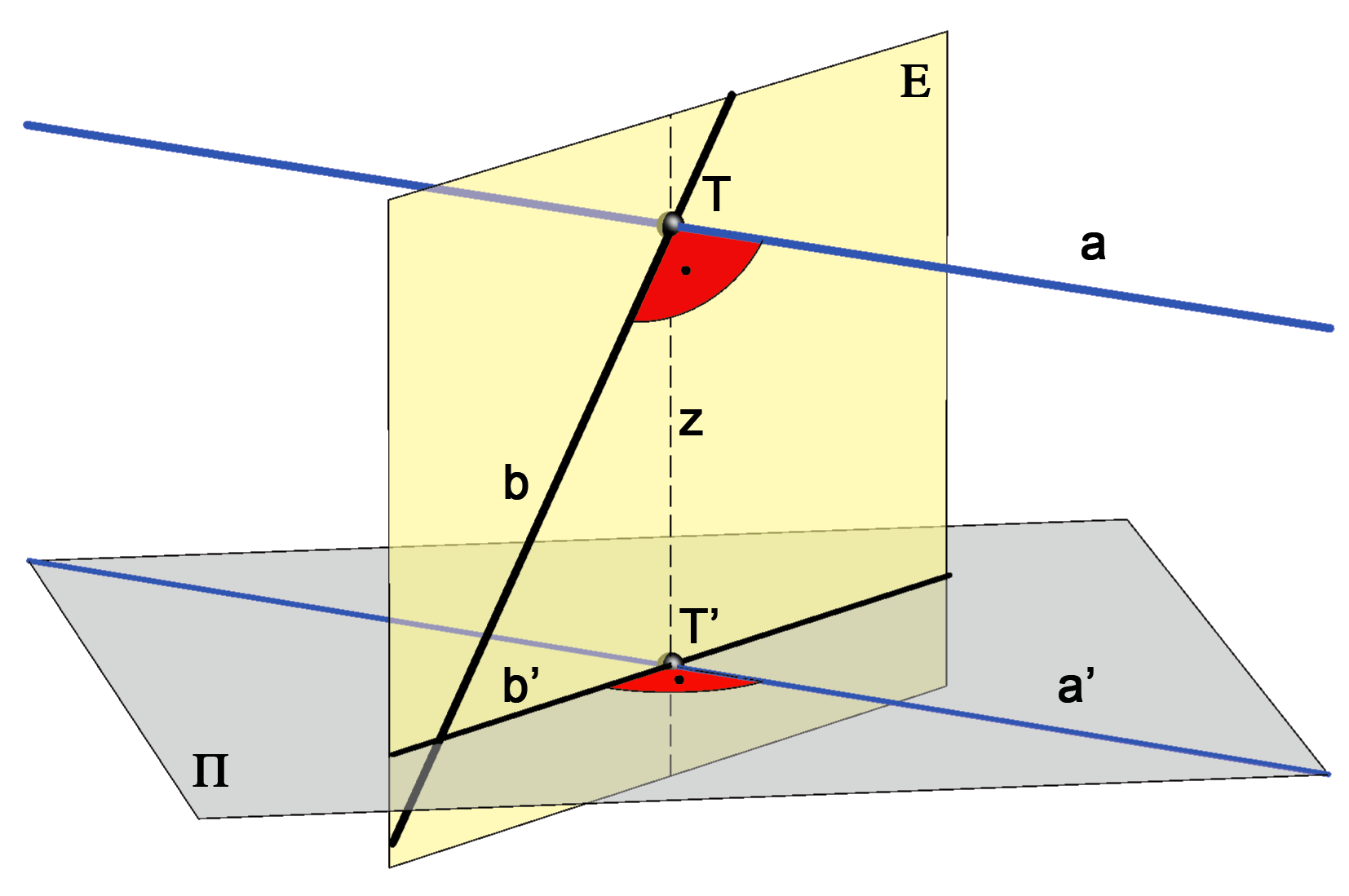A line a is parallel to the plane of projection Π and perpendicular to the line b. We need to prove that their projections are also perpendicular, i.e. a' ⊥ b'. A line a is perpendicular to the vertical projecting plane E that contains the line b. Namely, a is perpendicular to two lines in this plane: line b and the projection ray z. (Line a is parallel to the plane Π, while z is perpendicular to Π.) Since a' is parallel to a, it must be perpendicular to the plane E. Hence, a' is perpendicular to all lines in the plane E, as well as to the line b'. Proof can be written as: a ⊥ b & a | | Π=>a ⊥ E=>a' ⊥ E=>a' ⊥ b'.

### Straight line and a plane perpendicular to each other

Any line n perpendicular to the plane P is called a normal line of the plane P and their point of intersection, N = nP is called  pedal (perpendicular foot) of the line n.

• A line n is perpendicular to a plane P if and only if its horizontal projection (top view) is perpendicular to the horizontal trace of the plane and its vertical projection (front view) is perpendicular to the vertical trace of the plane, i.e.

nP<=>p'r1 & p''r2.

This claim follows from the fact that a line perpendicular to a plane must be perpendicular to all lines contained in that plane, also to its traces. Since the line is perpendicular to the horizontal trace r1 (r2) that belongs to the plane Π1 (Π2), horizontal (vertical) projection of this angle will be in true size - right angle.

The image below shows this statement and also shows those principal lines of the plane that intersect the normal line in its pedal point.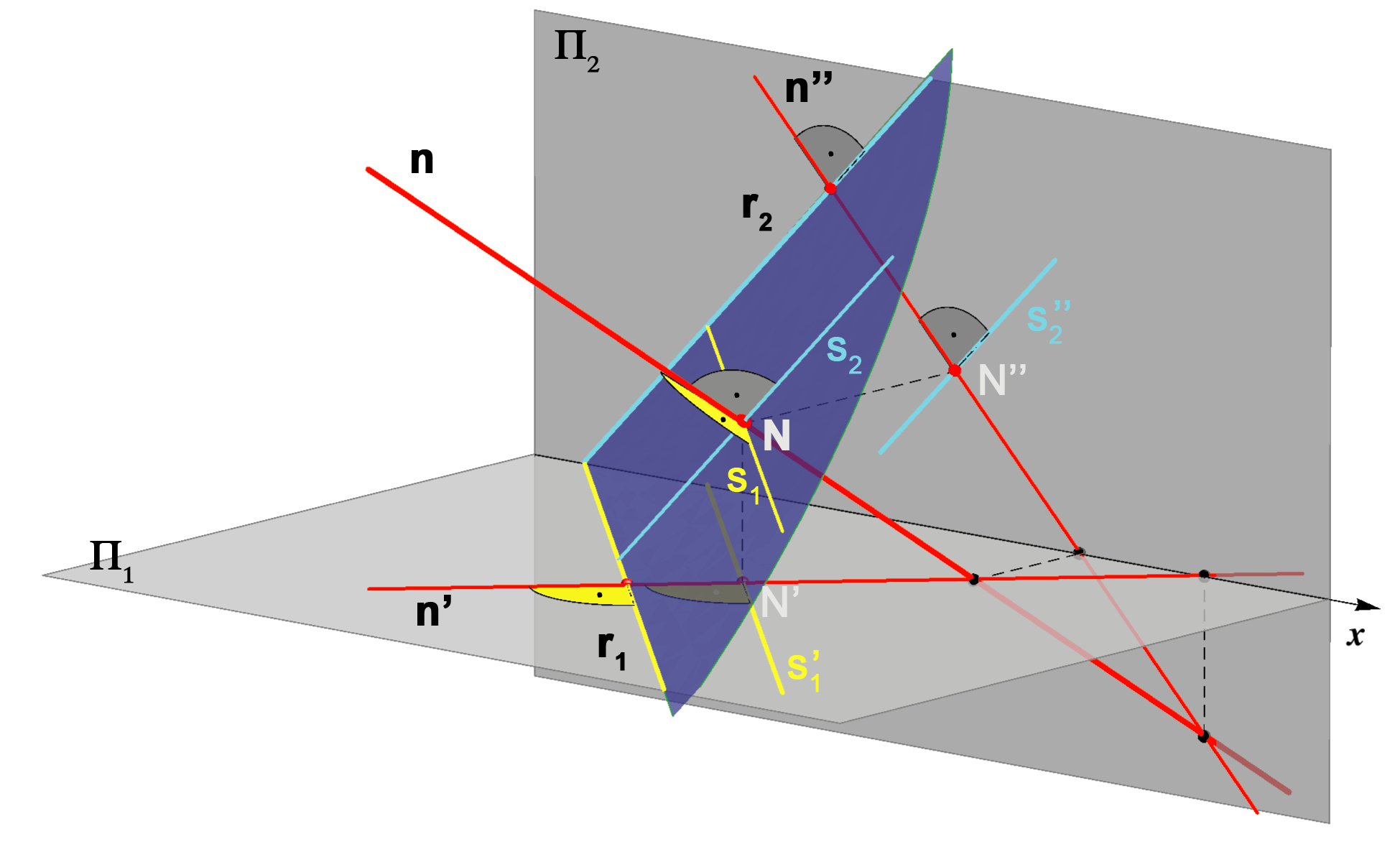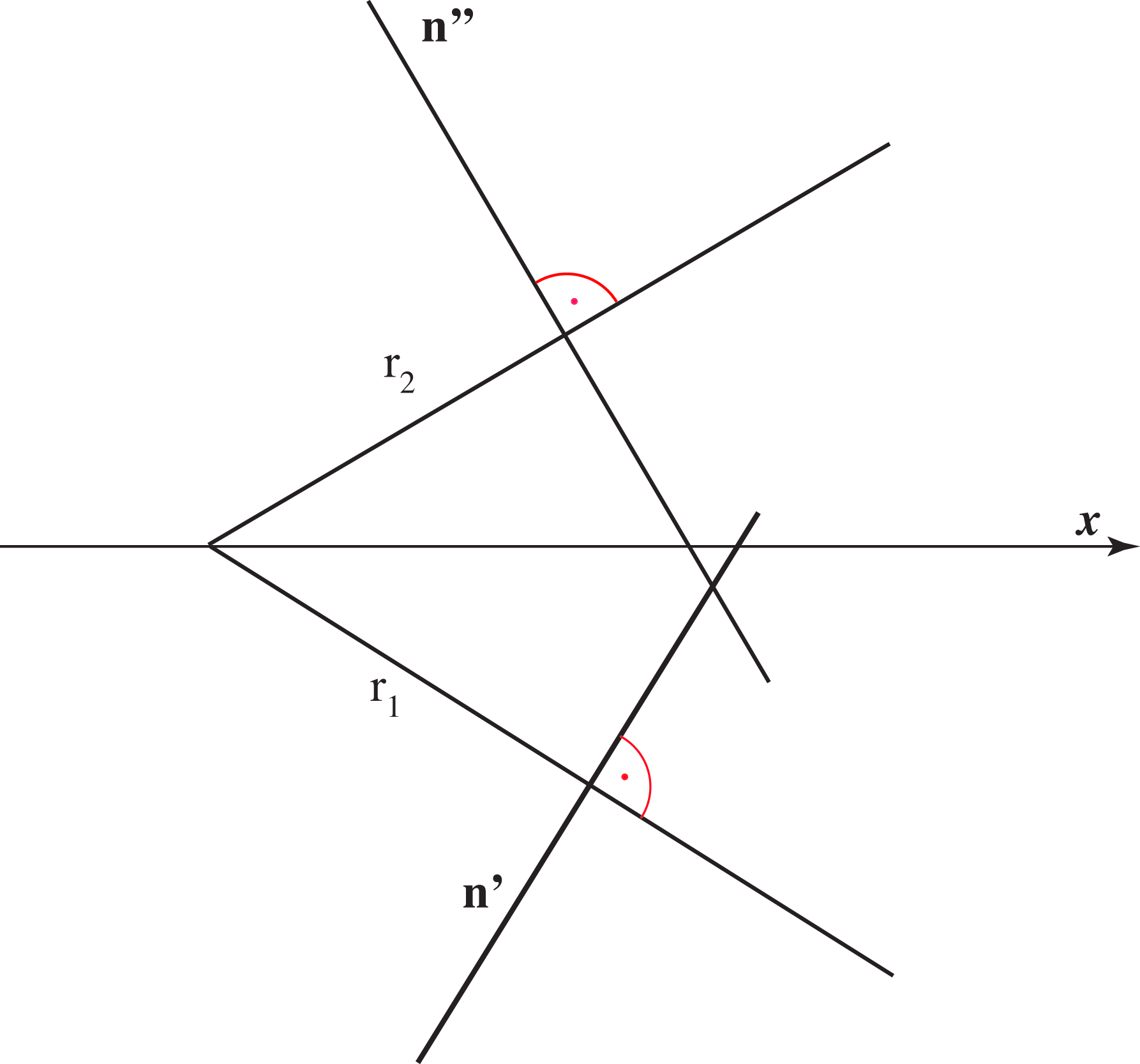Assignment 1: Construct the projections of a line that passes through the given point and it is perpendicular to the plane given by its traces.
Assignment 2: Construct the traces of a plane that passes through the given point and it is perpendicular to the given line.

Assignment 3: Determine the distance of a given point and a given plane (plane is given by its traces).
Assignment 4: Determine the distance between a given point and a given line.

### Bisector plane of a line segment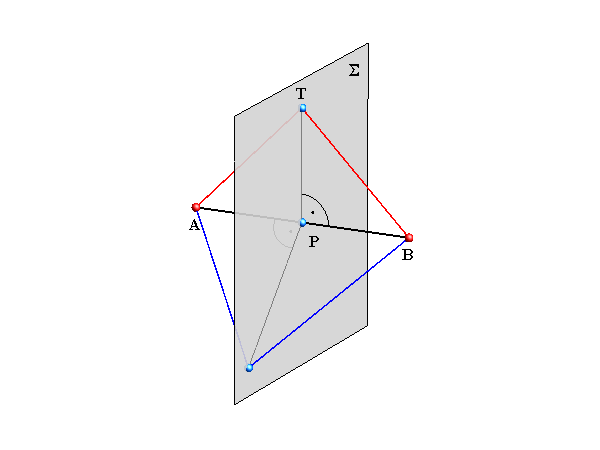T ∈ Σ<=>d(A,T) = d(B,T) Set of points that are equally distanced from points A and B belong to the plane called bisector plane of the line segment AB. The bisector plane of the line segment AB contains the midpoint of the line AB and it is perpendicular to the line AB.

Assignment 5: Construct the traces of the bisector plane of a given line segment.

### True length of the normal line

Let n be the normal line of the plane P and N their intersection point.
Let p1 be the 1st steepest line of the plane P that passes through the point N, and p2 the 2nd steepest line that passes through N.

Due to the property of orthogonal projection of the right angle mentioned before (n' ⊥ r1, n'' ⊥ r2), lines n and p1 will have the same top view (n' = p'1) and the lines n and p2 will have the same front view  (n'' = p''2).
Therefore, the lines n and p1 lie in the same horizontal projecting plane and the lines n and p2 lie in the same vertical projecting plane.

These properties are shown in the images below.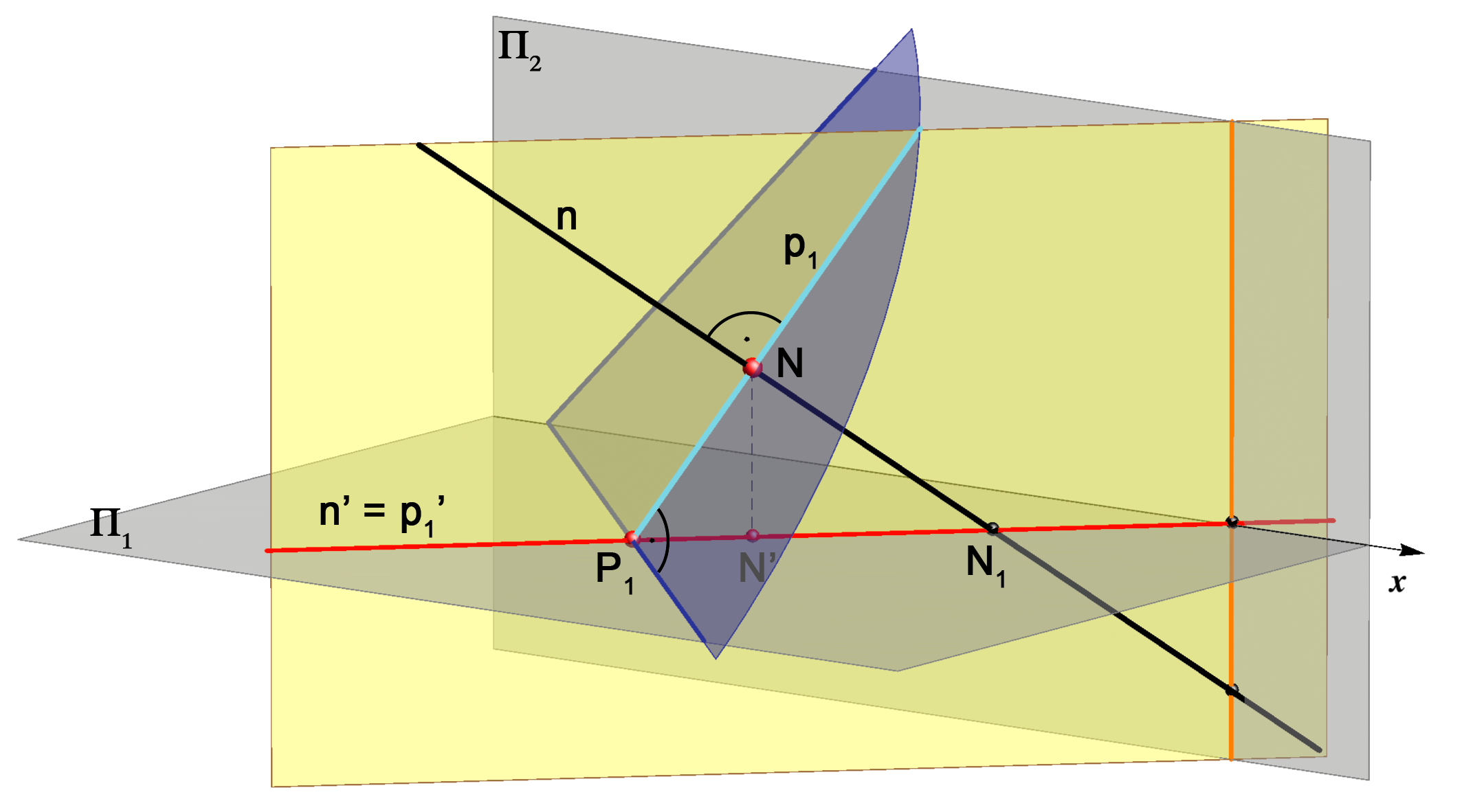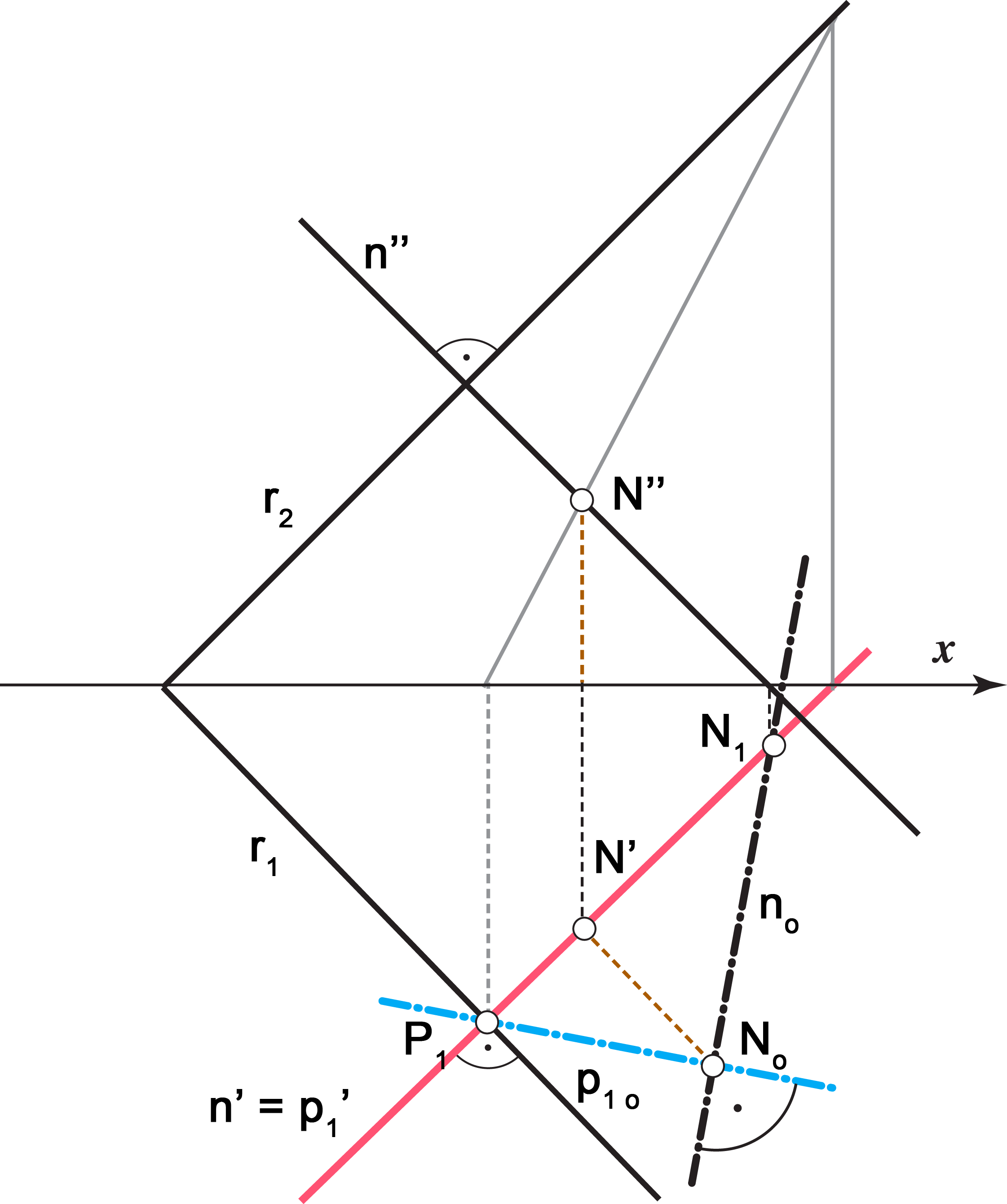The normal line and the1st steepest line through the pedal of the normal lie in the same horizontal projecting plane. The normal line rotated into the plane Π1 is perpendicular to the 1st steepest line rotated as well, they intersect at the projection of the pedal.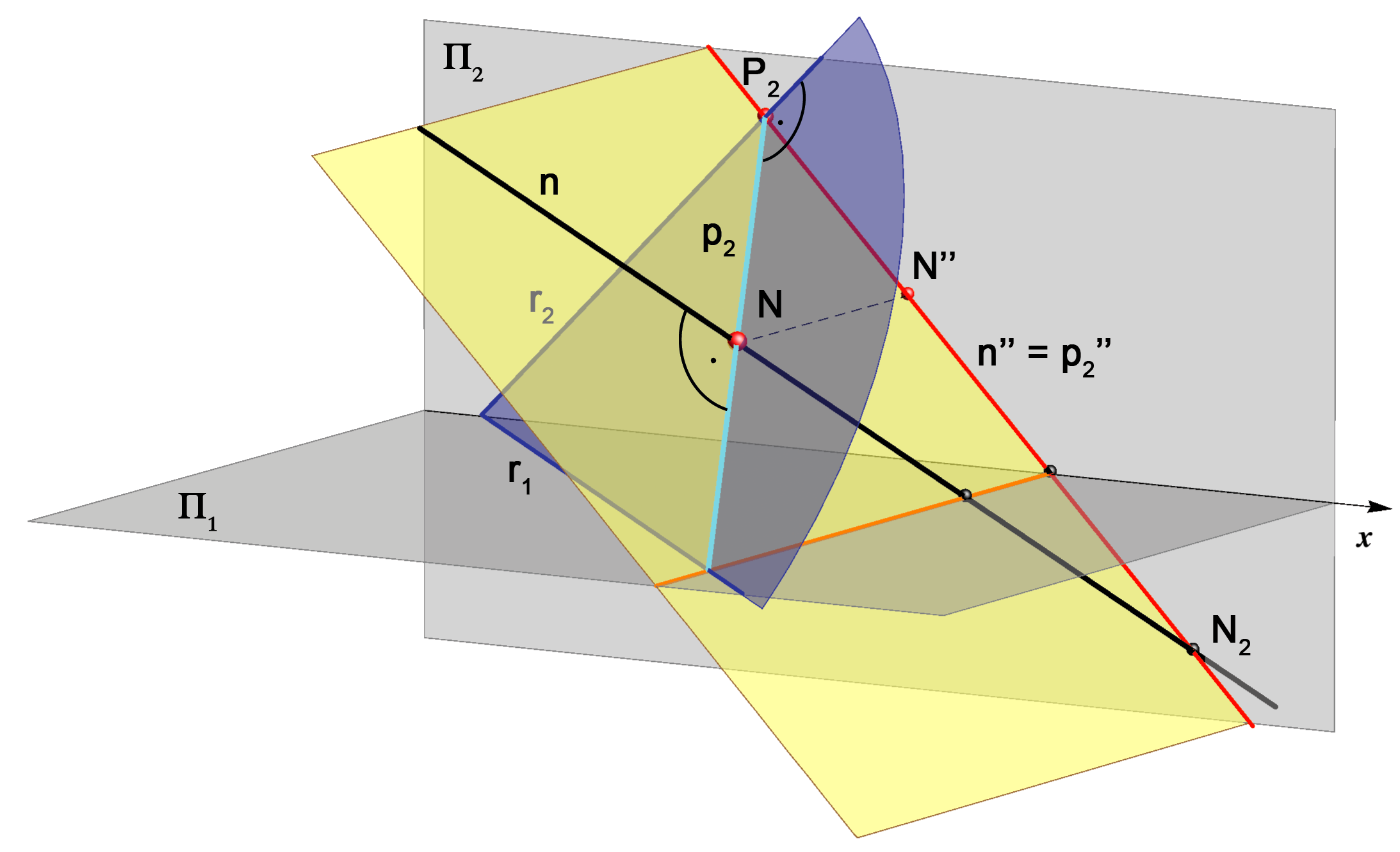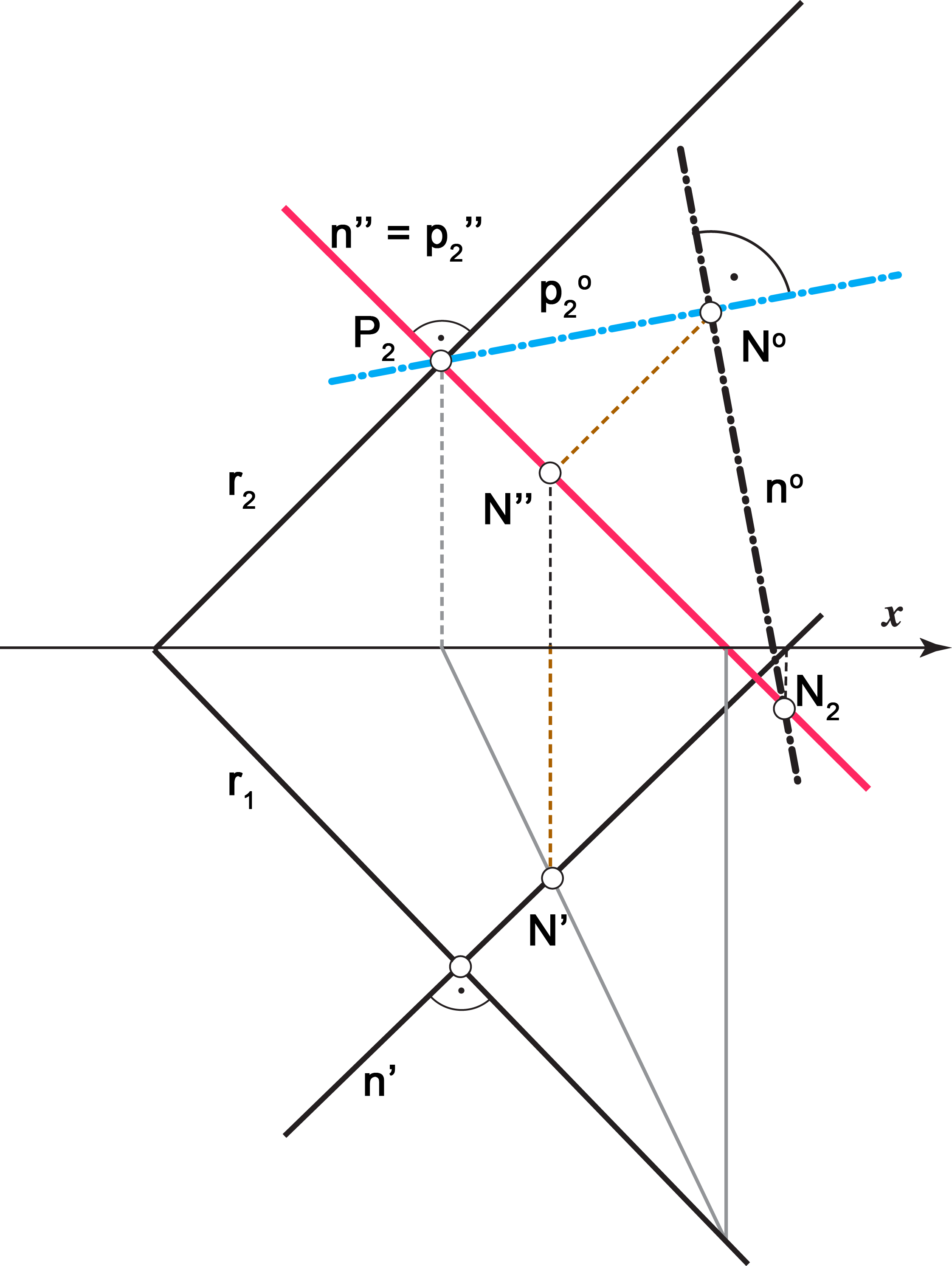The normal line and the 2st steepest line through the pedal of the normal lie in the same vertical projecting plane. The normal line rotated into the plane Π2 is perpendicular to the 2st steepest line rotated as well, they intersect at the projection of the pedal.

Assignment 6: Lay a line through the point T(1,3,–) that is perpendicular to the plane P(–3,4,3) and has length 4.

### Mutually perpendicular planes

• Two planes are mutually perpendicular if any one of these planes contain at least one line that is perpendicular to the other plane.

If two planes are mutually perpendicular then every plane contain infinitely many lines perpendicular to the other plane (pencil of perpendicular lines).

Using Monge's method it is NOT possible to determine whether two planes with given traces are perpendicular.
That is because perpendicular planes don't have perpendicular traces.
Try to visualize an example.

Assignment 7: Construct the traces of a plane through a given line that is perpendicular to a given plane.

Created by Sonja Gorjanc, translated by Helena Halas and Iva Kodrnja - 3DGeomTeh - Developing project of the University of Zagreb